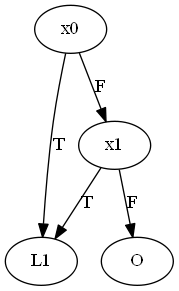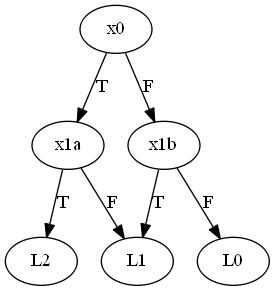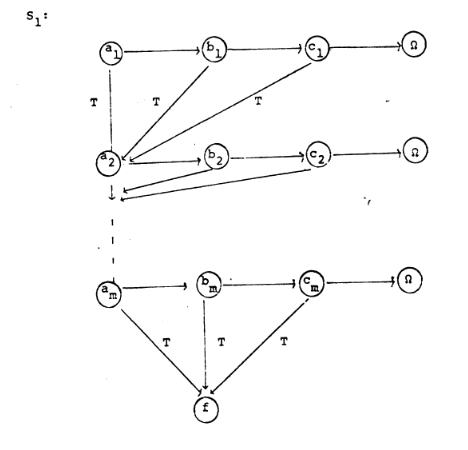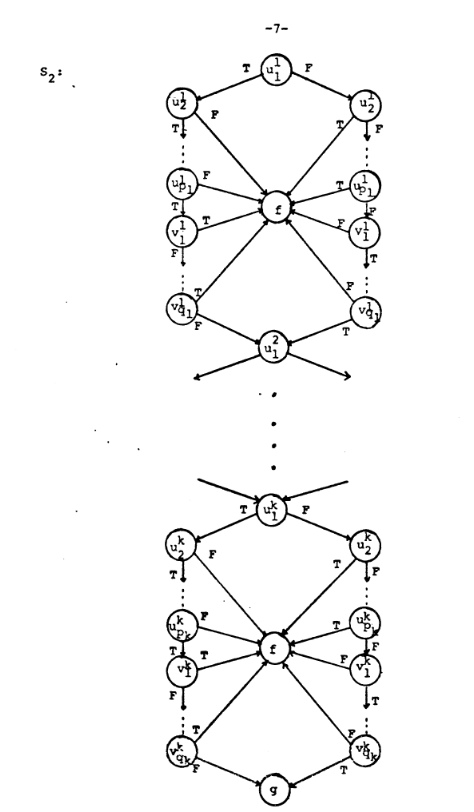Here is another scheme problem with a different kind of a reduction.

The problem: Non-Containment for Free B-Schemes. This is problem PO18 in the appendix.

The description: A “B-Scheme is” a rooted, directed, acyclic graph where each vertex has outdegree of either 0 (a “leaf”) or 2 (a “test”).  The test vertices label their out edges as “T” or “F”.  Each vertex gets a label.  Test vertices are labeled as boolean Boolean variables, leaves are labeled with function symbols (with the special symbol “Ω” meaning “undefined function”.

Multiple vertices can have the same label, but in a “free” B-scheme, no path from the root to a leaf hits two vertices with the same label.  We can “assign” truth values to the boolean variables, which then define a path through the scheme to a leaf.  The value of the truth assignment is the label given to the leaf we end up in.

Suppse we have two such B-Schemes, S1 and S2.  S1 is contained in S2 if for all assignments of variables, either S1 ends up in Ω, or S1 ends up in a leaf that is the same label as S2‘s leaf with the same assignment.

So, our question is: Given two free B-Schemes S1 and S2, is S1 not  contained in S2?

Example:

Here is our S1:“O” is the label for the Ω leaf

Here is S2(x1a and x1b both are labeled for the variable x1.  I had to give them different names so Graphviz didn’t think they were the same variable.)

S2 returns the number of true assignments of x0 and x1.  S1 correctly finds the same leaf if at least one variable is true, but goes to O otherwise.

But, S1 is NOT contained in S2 because assigning both variables to true puts S1 in L1 and S2 in L2.

Reduction: The paper by Fortune, Hopcroft, and Schmidt defines this problem and reduces from 3SAT. First, we replace all positive occurrences of the same variable xi with new variables ui1..uip (if it occurred p times).  We similarly replace all negative occurrences of xi with vi1..viq (if it appeared negated q times).  Our job is to build two schemes.

S1‘s job is to tell us if the formula is satisfiable.  Each clause (a1,b1, c1), gets a chain of vertices. It goes “across” on F, and down to the first variable of the second clause on T.  If all literals get assigned F, we end up in a Ω leaf.  The final clause has edges from T to our output “f” leaf (Yes, that means the vertex we reach when the formula is satisfiable and all of the clauses are true is labeled “f”.  I’m sorry.)  Here is the paper’s example.  Keep in mind that each of the a,b, and c vertices here represent literals whose actual value in the formula is one of the u and v variables we created above.S2‘s job is to make sure that all of our new u and v vertices have the same (and consistent) truth values.  If we ever have a ui with a different truth value than some other uj (or if it has the same truth value as its corresponding vj) we jump to f.  If everything is ok, we jump to a new end point g.  Here is the diagram from the paper.  Notice that they are doing this for all of the replacements for all of the variables in one scheme.If our original formula was satisfiable, we would find a way to make S1 go to f, and since all of the variable assignments are consistent, we could use those assignments to make S2 go to g, so S1 is not contained in S2,  In the other direction, if S1 is not contained in S2, then since all S1 can reach is Ω and f, it must be the case that there is an assignment that leads S1 to f and S2 to g.  S2 ends in g only when its u and v variables are assigned consistently.  S1 goes to f only when its clauses are all satisfied.  Thus, we have found a way to satisfy the formula.

Difficulty: 7.  This is different from the other scheme problems we have seen, where the second scheme is the trivial “yes” scheme.  Here, we need to use both schemes to check different aspects of the solution.  That’s pretty cool.